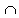Show that if more than half of the subsets of an n-element set are selected, then some two of the selected subsets have the property that one is a subset of the other.

SolutionShow that if more than half of the subsets of an n-element set are selected, then some two of the selected subsets have the property that one is a subset of the other.

I'll give two proofs of this fact.

(In the following |X| designates the number of elements in a finite set X, while 2X denotes the set of all subsets of set X.)

Select an arbitrary element x from a set A with n elements (n-set) and consider two families of subsets. The family N consists of all subsets of A that do not contain x; the family Y comprises the remaining subsets (each one of these contains x.) There are exactly 2n-1 subsets in both N and Y.

We start with a family B that contains more than a half of all subsets of A. By the Pigeonhole principle, one of the N or Y families contains at least a half of the subsets from B. If it's N, we may continue directly. If it's Y, we first note that all the subsets involved contain the chosen number x. If we drop it from all the subsets we arrive at exactly the same situation as in the first case. Let's summarize.

We are given a set Anew (this is the former A with the element x removed), for which set N (or Y with x removed) serves as the set of all subsets. Now, we know that |B| > |2A|/2 = |Anew|. But |B| = |NB| + |YB|. Therefore, either |NB| > |Anew|/2 or |YB| > |Anew|/2.

Depending on which of the inequalities holds, let Bnew denote either NB or YB with x removed. In either case, |Bnew| > |2Anew|/2. This is exactly our original problem, except that the set A now contains 1 element less than before.

We may now proceed either by induction (all it takes is to check some minimal set of a few elements) or by just regressing backwards to a set with a small number of elements, which amounts to the same thing. So let's consider a set with a small number of elements. How many should we take? Let's take n = 1. The set of all subsets of {1} consists of two sets - the empty set {} and {1}. To contain more than half of all subsets B is bound to contain both of them. In which case, it clearly contains two sets of which one {} is a subset of another {1}.

The following proof is by William A McWorter Jr..

Let again x be an element of the n-set A (the result is vacuously true when n=0). For each of the 2n-1 subsets A of A not containing x, form the pair {A, A∪{x}}. These pairs form a partition of the subsets of A. Now, given more than half of its subsets, some two must belong to the same pair, and so have the property that one is a subset of the other.

## Remark

The result obtained with the help of the Pigeonhole principle can be strengthened considerably: in order to assure that among selected subsets one contains another, one does not need to select more than half the subsets. Fewer will suffice.There is a very related problem:

Show that if more than half of the subsets of an n-element set are selected, there exists a pair of them such that one is not a subset of another provided none of the selected sets is empty.

Combine all subsets into pairs {X,Xc} of a set and its complement Xc = A - X. The number 2n-1 of such pairs is exactly half the number 2n of all subsets. Therefore, if more than half of the subsets are selected, there are two that fall into a single pair {X,Xc}. Since none of the selected sets is empty, neither X, nor Xc is empty. Hence neither contains the other.

## Example

One can't dispose of the prohibition to select an empty set. Indeed, the 2-set A = {1,2} has four subsets: Ø, {1}, {2}, {1,2}. One may select, for example, three subsets Ø, {1}, {1,2} such that they form an inclusion chain: Ø⊂{1}⊂{1,2}.

## Remark

Alternatively one may preclude selecting A itself.• 17 rooks on a Chessboard
• Chinese Remainder Theorem
• Pigeonhole in a Chessboard
• Pigeonhole in Concurrent Cuts
• Monochromatic Rectangle in a 2-coloring of the Plane
• Polynomial and Integer Division
• Power of three that ends with 001
• Pigeonhole Principle (subsets)
• Pigeonhole in Integer Differences
• Four Numbers, Six Differences, GCD of the Products
•